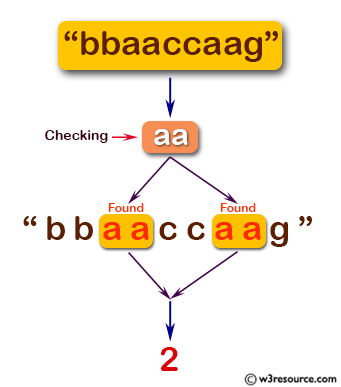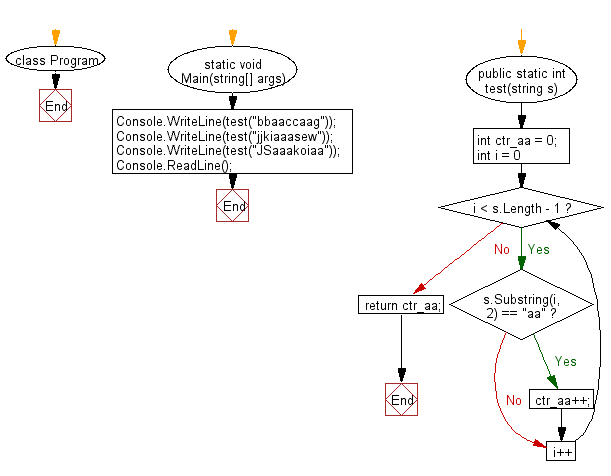﻿ C# - Count how many times 'aa' appears in a string# C# Sharp Basic Algorithm Exercises: Count the number of times the string 'aa' appears in a given string and assume that 'aaa' contains two 'aa'

## C# Sharp Basic Algorithm: Exercise-27 with Solution

Write a C# Sharp program to count the number of times the string "aa" appears in a given string and assume that "aaa" contains two "aa".

Pictorial Presentation:Sample Solution:-

C# Sharp Code:

``````using System;
namespace exercises
{
class Program
{
static void Main(string[] args)
{
Console.WriteLine(test("bbaaccaag"));
Console.WriteLine(test("jjkiaaasew"));
Console.WriteLine(test("JSaaakoiaa"));
}

public static int test(string s)
{
int ctr_aa = 0;
for (int i = 0; i < s.Length - 1; i++)
{
if (s.Substring(i, 2) == "aa")
{
ctr_aa++;
}
}
return ctr_aa;
}
}
}
```
```

Sample Output:

```2
2
3```

Flowchart:C# Sharp Code Editor:

Improve this sample solution and post your code through Disqus

What is the difficulty level of this exercise?

Test your Programming skills with w3resource's quiz.

﻿